Maths-
General
Easy

Question

# A Cylinder tank has a 75 cm diameter and 1.8 m height. Find the capacity in litres to three significant figures?Hint:

## The correct answer is: The required capacity is 795.536 L

### Explanations:Step 1 of 1:Given, diameter is 75 cm, so the base radius r = 75/2 cmHeight  h = 1.8 m = 180 cmCapacity of the tank = volume of the tank =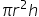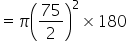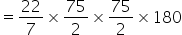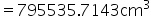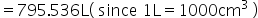Final Answer:The required capacity is 795.536 L#### With Turito Foundation.#### Get an Expert Advice From Turito.